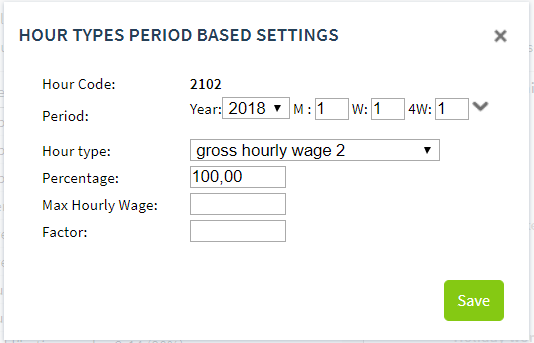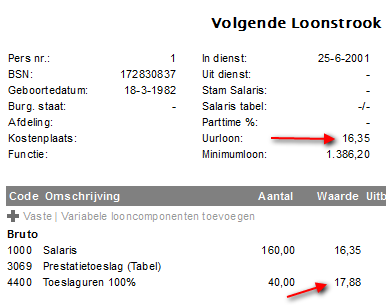Composed hourly wage (calculated hourly wage 2 or 3)

A combined hourly wage is an hourly wage that consists of different wage components. You can indicate which wage components these are.

Please note: the types of hours that you have given as hourly wage type 'calculated hourly wage 2 or 3' must have a higher wage code number than the wage code that you want to include in the calculation (in the example below, you can only make wage components count that are lower than output wage code W4400).

The calculated hourly wage is then the sum of all wage codes that you have included in the base of the combined hourly wage. You can calculate two combined hourly wages.

In the hour model, you can calculate an hourly code for this combined hourly wage. For this, you can use calculated hourly wage 2 (base 10302) or calculated hourly wage 3 (10303)berekend uurloon 3 (grondslag 10303) :Go to the wage model and select the wage codes that you want to combine for the combined hourly wage. Add bases for each wage code; choose base 10302 or 10303 for this:

The hourly wage used to make calculations now has a different value on the payslip than the calculated hourly wage:Retirement & supplementary payment using hour types "calculated hourly wage 1 or 2"

This does not work. If an employee is retired, then it is no longer possible to work with the following dashlets:

• Salary
• Fixed wage components

Because calculated hourly wage 1 or 2 deals with the hourly wage from the Salary dashlet, this combination is not possible.

There are two possible solutions:

• You process these hours within the period type during which the employee is still working (final period); or
• you work out the amount and enter this as a one-time wage component.
Follow